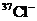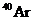Chapter 4, Problem 89APIntroductory Chemistry: A Foundati...

9th Edition
Steven S. Zumdahl + 1 other
ISBN: 9781337399425

Solutions

Chapter
SectionIntroductory Chemistry: A Foundati...

9th Edition
Steven S. Zumdahl + 1 other
ISBN: 9781337399425
Textbook Problem

Which of the following is(are) true regardingandsame group number on the periodic table same number of protons same number of neutrons same number of electrons

Interpretation Introduction

Interpretation:

The true statement should be identified.

Concept Introduction:

The atomic number of an atom specifies the number of protons and number of electrons in a neutral atom. Number of neutrons can be calculated with the help of mass number because mass number is equal to sum of number of electrons and protons.

Explanation

Reason for correct option: Chlorine has 17 electrons and 17 protons whereas Cl- ion has 17+1 = 18 electrons as it has -1 charge...

Still sussing out bartleby?

Check out a sample textbook solution.

See a sample solution

The Solution to Your Study Problems

Bartleby provides explanations to thousands of textbook problems written by our experts, many with advanced degrees!

Get Started

T-cells are immune cells that "read" and "remember" chemical messages to identify future invaders. T F

Nutrition: Concepts and Controversies - Standalone book (MindTap Course List)

Match the concepts:

Human Biology (MindTap Course List)

What impact has recombinant DNA technology had on genetics and society?

Human Heredity: Principles and Issues (MindTap Course List)